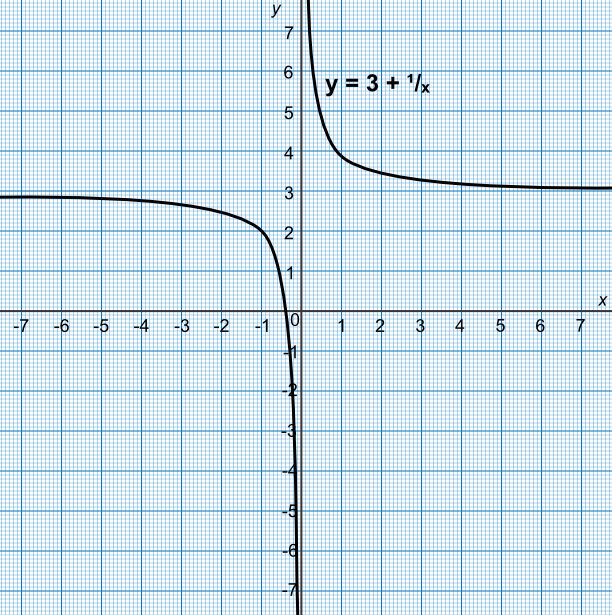Graphing Reciprocal Functions

## Graphing Reciprocal Functions

A reciprocal function, such as y = (1)(x), creates two curves on the graph in opposite quadrants. There are values on the graph which cannot be found: x = 0 is a division by zero; and y = 0 requires x to be equal to infinity. The values of x = 0 and y = 0 (in this example) are known as asymptotes: these are the lines that the curves tend towards, but never touch.

## Example 1

The function y = 3 + frac(1)(x) has been plotted on the graph below.

What are the asymptotes for this graph?The value y = 3 requires x to be set to infinity.

The value x = 0 is a division by zero.

Answer: x = 0 and y = 3

## Example 2

Using the graph, above, estimate the value of y when x = -1.

Check the answer by substituting into the equation: y = 3 +frac(1)(-1) = 2.